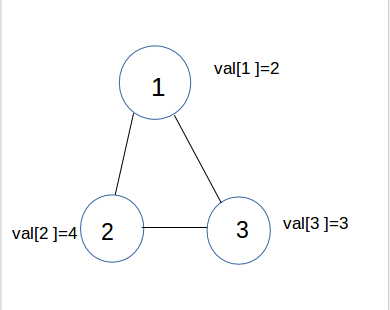Learning Graph
Tag(s):

## Algorithms, Easy, Graph, Graph Representation, Graph Theory

Problem
Editorial
Analytics

Monk once went to the graph city to learn graphs, and meets an undirected graph having N nodes, where each node have value $val[i]$ where $1 \le i \le N$. Each node of the graph is very curious and wants to know something about the nodes which are directly connected to the current node.

For each node, if we sort the nodes (according to their values), which are directly connected to it, in descending order (in case of equal values, sort it according to their indices in ascending order), then what will be the number of the node at $k^{th}$ position, if positions are given starting from 1.

Now Graph gave this task to Monk. Help Monk for the same.

Input Format :

The first line will consist of 3 space separated integers N,M, k denoting the number of nodes, number of edges and value of k respectively.
In next line, there will be N space separated integers, $val[ i ]$ , where $1 \le i \le N$, denoting the value of $i^{th}$ node.
In next M lines, each line contains 2 integers X and Y, representing an edge between X and Y.

Output Format
Print N lines, where $i^{th}$ line contains the required node for the $i^{th}$ node. If there is no such node, print -1.

Constraints: :

$1 \le N \le 10^3$
$1 \le M \le 10^6$
$1 \le k \le M$
$1 \le val[ i ] \le 10^4$
$1 \le X,Y \le N$

SAMPLE INPUT
3 3 2
2 4 3
1 3
1 2
2 3

SAMPLE OUTPUT
3
1
1

ExplanationThe node with $2^{nd}$ largest value from all of the adjacent nodes of node 1 is node 3.
The node with $2^{nd}$ largest value from all of the adjacent nodes of node 2 is node 1.
The node with $2^{nd}$ largest value from all of the adjacent nodes of node 3 is node 1.

Time Limit: 1.0 sec(s) for each input file.
Memory Limit: 256 MB
Source Limit: 1024 KB
Marking Scheme: Marks are awarded when all the testcases pass.
Allowed Languages: Bash, C, C++, C++14, Clojure, C#, D, Erlang, F#, Go, Groovy, Haskell, Java, Java 8, JavaScript(Rhino), JavaScript(Node.js), Julia, Kotlin, Lisp, Lisp (SBCL), Lua, Objective-C, OCaml, Octave, Pascal, Perl, PHP, Python, Python 3, R(RScript), Racket, Ruby, Rust, Scala, Swift, Swift-4.1, TypeScript, Visual Basic

## CODE EDITOR

Initializing Code Editor...

## This Problem was Asked inChallenge Name

CodeMonk (Graph Theory Part I)

OTHER PROBLEMS OF THIS CHALLENGE
• Algorithms > Graphs
• Algorithms > Graphs
• Algorithms > Graphs
• Algorithms > Graphs